SVG Math Animation Example: Trammel of Archimedes – Ellipse Tracing Mechanism

March 22, 2010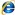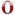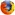http://www.bogotobogo.com
http://www.epicmath.com

SVG Math Animation Example: N-Cusp Hypocycloid with Javascript

March 22, 2010http://www.bogotobogo.com
http://www.epicmath.com

SVG Math Animation Example: Pythagorean (Pythagoras’) Theorem – SMIL Proof #2

March 22, 2010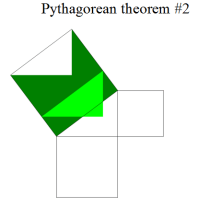http://www.bogotobogo.com
http://www.epicmath.com

SVG Math Animation Example: Irrational Epicycloid with Javascript

March 20, 2010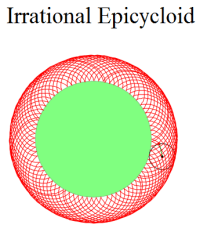http://www.bogotobogo.com
http://www.epicmath.com

SVG Math Animation Example: N-Cusp Epicycloid with Javascript

March 20, 2010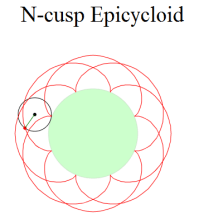http://www.bogotobogo.com
http://www.epicmath.com

SVG Math Animation Example: Cycloid with Javascript

March 20, 2010http://www.bogotobogo.com
http://www.epicmath.com

SVG Animation Example: Pythagorean (Pythagoras’) Theorem – SMIL

March 19, 2010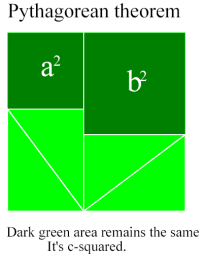SVG Animation Example: Nested Apollonian Gasket

March 18, 2010

SVG Animation Example: Nested Apollonian Gasket [n,n+1,n(n+1),n(n+1)+1] (n=2,10) – Soddy (three tangential Circles) and Descartes’ theorem. It may be slow because it’s calculating circles on the fly and my circle packing algorithm is not efficient.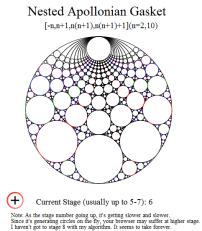http://www.bogotobogo.com
http://www.epicmath.com

SVG Animation Example: Apollonian Gasket (-15,32,32,33)-Core section

March 17, 2010

SVG Animation Example: Apollonian Gasket (-15,32,32,33)-Core section: Soddy (three tangential Circles) and Descartes’ theorem. It may be slow because it’s calculating circles on the fly.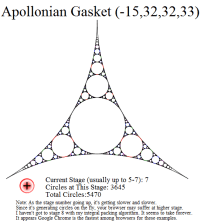http://www.bogotobogo.com   http://www.epicmath.com

SVG Animation Example: Apollonian Gasket (-15,32,32,33)

March 17, 2010

SVG Animation Example: Apollonian Gasket (-15,32,32,33)- Soddy (three tangential Circles) and Descartes’ theorem. It may be slow because it’s calculating circles on the fly.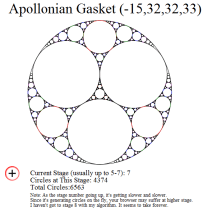http://www.bogotobogo.com   http://www.epicmath.com